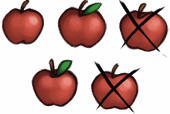Definition of

SubtractTo take one number away from another.

If we have 5 apples and then subtract 2 we are left with 3.

The symbol of subtraction is −

Example: 5 − 2 = 3